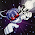## Dienstag, 21. Mai 2013

### Parallel all permutations - non-recursive - GPU optimal

This post is a general one - not voxel related.

Some time ago I developed a a simple, fast and yet non-recursive method to directly compute the n'th permutation. Thats useful especially when working on the graphics card with CUDA for parallel evaluations. (it might have been published somewhere already - so if you know the reference, you can post it as a comment)

The algorithm is based on the following property. Lets say there are 6 characters in the string to permute. Then the result is 6! = 720 combinations. In the first column of the results, we get 720/6=120 lines of each character. This means if the index counts from 0 to 719, then the first character to be removed is computed as remove_index = index/120.

For the next column, 5 numbers are left. This means, that 120 = 5 * 24 lines of the same character - and so on.

We the following list of removes:
column0: remove_index = (a/120)%5;
column1: remove_index = (a/24)%4;
column2: remove_index = (a/6)%3;
column3: remove_index = (a/2)%2;
column4: remove_index = (a/1)%1;

Code snipplet:

std::string default = "12345";
int perm=1, digits=default.size();
for (int i=1;i<=digits;perm*=i++);
for (int a=0;a
<perm;a++)
{
std::string avail=default;
for (int b=digits,div=perm;b>0; b--)
{
div/=b;
int index = (a/div)%b;
printf("%c", avail[index] );
//avail[index]=avail[b-1]; // non-lexigraphic but fast
avail.erase(index,1) ; // lexigraphically correct
}
printf("\n");
}
printf("permutations:%d\n",perm);

In the code, perm defines the Lehmer code (wiki)

#### Kommentare:

1.Can you give us an example of a more practical use for your algorithm, please?

2.It has multiple applications that range from cryptography over group theory (math), randomization to the travelling salesman problem

3.Writing this just before going to bed (and thus implying an excuse for not really understanding your code snippet one bit atm :P). But I'd say it looks really, really brilliant!

I made a similar one a while (well, years) ago. Not so elegant or short, but it does the same thing - outputs a particular permutation for a string directly (Or actually not a string per se, but a character position remapping array). Just input the string and which permutation nr. The last permutation is the input string in reverse.

Code snippet (obviously I'm not as versed in C++ as you):

// Make combination nr. by mapping letter positions to p[]
// Combination nr. 1 is original string
// last combination is original string backwards

// nr = combination (or permutation) nr to return.
// a = nr of characters in the string
// p[x] = string remapper

// p[x] = new x.th letter position from position p[x] in source string
// ex: p = 3 means new first character is the old 3rd character (from original string).

void shuffle(long nr, int a, int p[])
{
int x = a;
int s;
int y;
do // magic
{
s = a - x; // shuffle in reverse (more natural)
p[s] = (int)((nr-1)/fac((long)x-1))+1;
nr = nr - (fac(x-1) * (long)(p[s]-1));
x--;
} while (x>0);
x=a-1;
do // more magic
{
y = x - 1;
do
{
if (p[y]<=p[x]) p[x] = p[x] + 1;
y--;
} while (y>=0);
x--;
} while (x>0);
}

(Probably the "shuffle" function should have been named "permute").

Regards,
raron

4.faster version here which also evaluates

https://sites.google.com/site/cudapermutations/

1.Thank you for the reference. Does it have a lower complexity or is it just faster due to GPU?